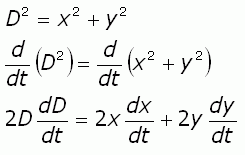SEARCH HOMEMath Central Quandaries & QueriesQuestion from Alexis, a student: Example 1. An observer is tracking a small plane flying at an altitude of 5000 ft. The plane flies directly over the observer on a horizontal path at the fixed rate of 1000 ft/min. Find the rate of change of the distance from the plane to the observer when the plane has flown 12,000 feet after passing directly over the observer.Alexis,

You can probably see right away that you have a right triangle. So start with some labels: y for the vertical distance above the observer, x for the horizontal distance from the observer and D for the distance along the hypotenuse.

You know that y is constant, so dy/dt is 0. You know that dx/dt is 1000 ft/min. You know that at all times, pythagoras' theorem applies, so for any particular given value of x (x = 12000 ft), you can calculate D by using Pythagoras.

As well, start with Pythagoras and differentiate both sides of the equation, using the chain rule:You know all these terms except dD/dt which is the rate of change of the distance between the observer and the plane, the amount you are asked to find. So solve for dD/dt, substitute in the known values and you'll have completed the problem.

Cheers,
Stephen La Rocque.Math Central is supported by the University of Regina and The Pacific Institute for the Mathematical Sciences.